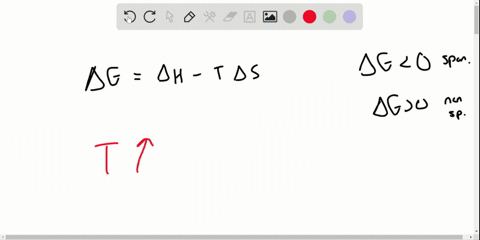Enroll in one of our FREE online STEM summer camps. Space is limited so join now!View Summer Courses### With its components in their standard states, a c…

01:29Ohio State University

Need more help? Fill out this quick form to get professional live tutoring.

Get live tutoring
Problem 48

Explain your answers to each of the following for the change depicted below. (a) What is the sign of $\Delta H_{\text { sys }} ?(b)$ What is the sign of $\Delta S_{S y s} ?(c)$ What is the sign of $\Delta S_{\text { surr }} ?(\text { d) How does the }$ sign of $\Delta G_{\text { sys }}$ vary with temperature?

Check back soon!

## Discussion

You must be signed in to discuss.

## Video Transcript

were given a diagram of a chemical reaction, and we want to you answer for different questions about this regarding the signs of the different properties of chemical variables associated with this reaction and part A. We want to determine what the sign of the change in M pulpy Delta h of the system will be. Remember that when we're considering the sign of Delta H. It positive value value indicates an endo thermic reaction, meaning that we have to put heat into the system in order to drive it. And a negative value means that it is an exo, thermic reaction that gives off heat to the surroundings. If we see if we look at the diagram, we see that we have 16 individual green Adams here, and after the reaction, we have eight hairs. Of those two green Adams bonded together. Whenever we form bonds, we release energy. If we think about formation reactions, for example, of forming a compound from its elements such as solid carbon was oxygen gas, which occur naturally to form a co two gas. We know that the delta H of formation value at standard conditions for that will will be less than zero were negative. His energy is always given off and released when bonds form. So therefore, for part A he dealt to each of the system is less than zero. This is an exo thermic reaction due to the formation of bonds or B. We want to determine how the entropy of the system is affected. We look, we see that we go from 16 gas particles down to eight gas particles. We become more ordered after the reaction, since in the products we have to two of those original articles bonded together, we form bonds and the total number of gas molecules decrease and gases of the greatest entropy out of the three states of matter. So if we have fewer gasses after the reaction compared to before, and we have more order after the reaction meaning that the entropy decreased in order to get the entropy of the system to decrease, that means that it has to have a a negative value less than zero. And now we want to determine the sign of Delta s of these surroundings. Remember that from part A. We can say that this is an exo thermic reaction that releases energy due to bond formation and therefore the surroundings increase in temperature. As we increased temperature, we increase entropy. And so since the surroundings are getting harder, that means that the entropy of the surroundings is increasing, so that would come out to a positive value. Finally, in part to you. We want to determine how the change in Gibbs free energy of the system is affected by changes in temperature. We use this equation to help us determine that Delta G equals still to age minus T Delta s We know from part A a Delta H is negative. We know from part B A. Delta s is negative if we were to increase the temperature, which is temperature is always in in this case and given and units of absolute temperature. Kelvin. So it's always going to be positive if we increase the temperature continually to a high positive value and we subtract it with that negative value for Delta S in this product is going to be negative. And when we subtract that negative value from the negative Delta H, that temperature is high enough, it will eventually overcome this this negative delta each value to become positive. So That means that Delta G will eventually become positive at high temperatures and in the opposite would also be true that that is, that decreasing the temperature would lead to negative values of Delta G and therefore make the reaction spontaneous. So to rate that out increase in temperature will lead to an increase in Delta G. Make it non spontaneous once it becomes positive and a decrease in temperature will lead to a decrease in Delta G and make the reaction become more spontaneous as a Delta G becomes negative.